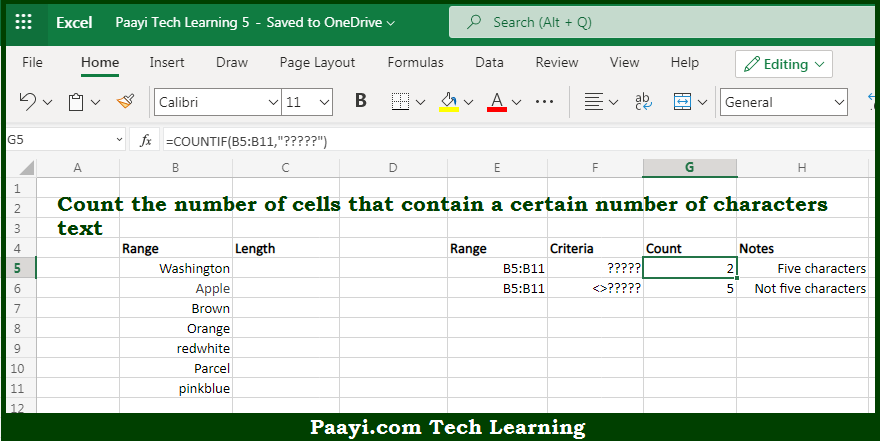# Learn How to Count Cells that Contain Five Characters in Microsoft Excel

Written by | 0 Comments | 449 Views

In this article, you will learn how to COUNT various things in Microsoft Excel using a single or combination of functions and its purpose in Microsoft Excel. You will also get to know how to count cells that contain five characters.

Count Cells that Contain Five Characters in Microsoft Excel

The main purpose of this formula is to count the number of cells that contain a certain number of characters in text. Here we will learn how to count cells having five text characters in Microsoft Excel.  That implies, with the help of a formula based on the  COUNTIF function you can able to count the number of cells that contain a certain number of characters. It should be noted that the generic form of the formula provided below, "rng" is a range of cells, and "?" is a wildcard matching any single character. So, with the help of this formula, you can able to count cells containing five text characters.

General Formula to COUNT Cells that Contain Five Characters

=COUNTIF(rng,"?????")

The Explanation for the COUNT Cells that Contain Five CharactersSo we know that with the help of the given formula above you can able to count the number of cells that contain a certain number of characters text. Here we will learn how to count cells having five text characters in Microsoft Excel. As we know that, when you use the COUNTIF function is used to count the number of cells in the range that contain five characters by matching the content of each cell against the pattern "?????".

It is supplied as the criteria for the COUNTIF function. The  "?" symbol is a wildcard in Microsoft Excel that means "match any single character". That means this pattern will count cells that contain any five characters. The result is returned as a number if the count of cells matches this pattern. So, with the help of this formula, you can able to count cells that contain five characters.